# Themes, topics - math word problems

#### Number of problems found: 925

• Pumps 3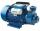Two pumps of the same power fill the garden pool for 10 hours. How many of these pumps would have to use if we want to shorten the filling of the pool to four hours?
• Master and apprentice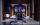Master painted the roof in 3 hours and apprentice for 4 hours. How many of roof they painted in hour and how many in three quarters of an hour?
• Train speedTwo guns were fired from the same place at an interval of 10 minutes and 30 seconds, but a person in a train approaching the place hears second shot 10 minutes after the first. The speed of the train (in km/hr), supposing that sound travels at 340 m/s is:
• Resultant forceCalculate mathematically and graphically the resultant of a three forces with a common centre if: F1 = 50 kN α1 = 30° F2 = 40 kN α2 = 45° F3 = 40 kN α3 = 25°
• Fertilizer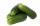Mr. Gherkin use for fertilization 7% solution of fertilizer. 17 liters of it still left. How much water must be added to the solution to make only 3% solution?
• Clock Tower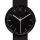What angle is betwenn hands on Clock Tower when show 17 hours and 35 minutes?
• Cottages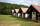The summer camp is 41 cottages. Rooms are for three and for four in them. How many of the 140 campers lives of three?
• CandyThe price for 1kg of more expensive candy is 125 CZK. The price for 1kg of cheaper candy is 100 CZK. We make two different blends of candy. And now. The second blend contains 2kg of more expensive candy and several kg of cheaper candy. The price per 1 kg
• Working togetherOne painter paint the nursery in 10 days, the second in 8 days. How many days will the nursery be painted if they work together?
• The tram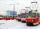The tram is moving with acceleration a = 0.3m/s2. How long it will pass the first meter of track? How long does it take 10 meters. What is its speed at the end of the 10 meters track?
• Two diggers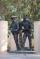There are two diggers. One digger digs a pit 46 hours second gidding 2 times faster. a) how long took to dig a pit second digger b) how long took dig together
• Steamship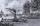Between the two ports on the river is going steamship. The journey there and back takes 8:42. Downstream steamship traveling at 20 km/h, upstream 9 km/h. What is the distance between the ports?
• One million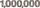Write the million number (1000000) by using only 9 numbers and algebraic operations plus, minus, times, divided, powers, and squares. Find at least three different solutions.
• Otto and Joachim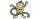Otto and Joachim go through the woods. After some time Otto tire and make 15 minutes stop. Joachim meanwhile continues at 5 km/h. Otto when he set off again, first running speed of 7 km/h, but it keep only 30 sec and 1 minute must continue at 3 km/h. This
• Tractors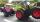Two tractors plow the field in 4 hours together. If the first tractor plow half of the field and then the second tractor completed the job, it would take 9 hours. How many hours does the field plow for each tractor separately?
• Vinegar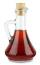What percentage vinegar we get if we mix 1 if dm³ eight percent vinegar with 1.5 dm³ six percent vinegar?
• Ruler and compassUse a ruler and compass to construct a triangle ABC with AB 5cm BAC 60° and ACB 45°.
• TruckIn 7 hours started from town Krnov truck at speed 40 km/h. Passenger car started against it in 8 hours 30 minutes from the city of Jihlava at speed 70 km/h. Distance between this two cities is 225 km. At what time and at what distance from Krnov this two
• Machines 2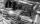2 machines for 50 hours produces 2,000 products. How many machines need to buy to make in 30 hours 15,000 products?
• TrianglesIvo wants to draw all the triangles whose two sides of which have a length of 4 cm and 9 cm and the length of the third side is expressed in whole centimeters. How many triangles does he have?

Do you have an interesting mathematical word problem that you can't solve it? Submit a math problem, and we can try to solve it.

We will send a solution to your e-mail address. Solved examples are also published here. Please enter the e-mail correctly and check whether you don't have a full mailbox.

Please do not submit problems from current active competitions such as Mathematical Olympiad, correspondence seminars etc...